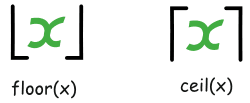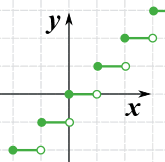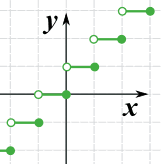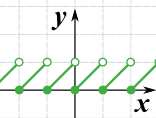# 向上取整与向下取整函数

### 例子：2.31 向上取整和向下取整是多少？2.31 向下取整是 2
2.31 向上取整是 3

5 向下取整是 5
5 向上取整是 5

x 下取整 上取整
−1.1 −2 −1
0 0 0
1.01 1 2
2.9 2 3
3 3 3

## 符号## 定义

### 例子：2.31 向下取整的正式定义

…… 要小于（或等于） 2.31，对不？

• 2 是小于 2.31 ……
• 1 也是小于 2.31。
• 以下的也是：0-1、-2、-3等等

## 图（注意：实心圆点的意思是 "包括"## "Int" 函数

"Int" 函数（英语 "integer" 的简写）和 "向下取整"函数一样，但当输入为负数时，有些计算器和电脑程序返回不同的答案：

• 有些是这样：int(−3.65) = −4（和向下取整函数一样）
• 另外一些是这样：int(−3.65) = −3（规则是：在旁边最接近零的整数，即是 "扔掉 .65"）

## "Frac" 函数

frac(x) = x − floor(x)Frac 函数

### 例子：frac(3.65) 是什么？

frac(x) = x − floor(x)

### 例子：frac(−3.65) 是多少？

frac(x) = x − floor(x)

• 有些说：frac(−3.65) = 0.35，即是 −3.65 − (−4)
• 有些说： frac(−3.65) = −0.65，即是 −3.65 − (−3)